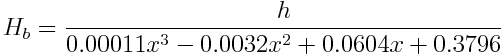# Details for predicting adult heights

The formulas used here are taken from the book Go Figure!: Using Math to Answer Everyday ImponderablesHeight for girls is predicted bywhere h is the current height and x is the current age.

For boys the corresponding formula isThese formulas assume that girls reach their adult height at around 13.5 years and that boys reach their adult height around 16.5 years.

Of course these are only predictions based on averages. Individuals may not follow the predictions of the formula.